# 7.10.3 The Addition and Subtraction of Coloured Lights (Structured Questions)

Question 1:
Diagram 1.1 and Diagram 1.2 show an experiment to study the effect of coloured filter on white light.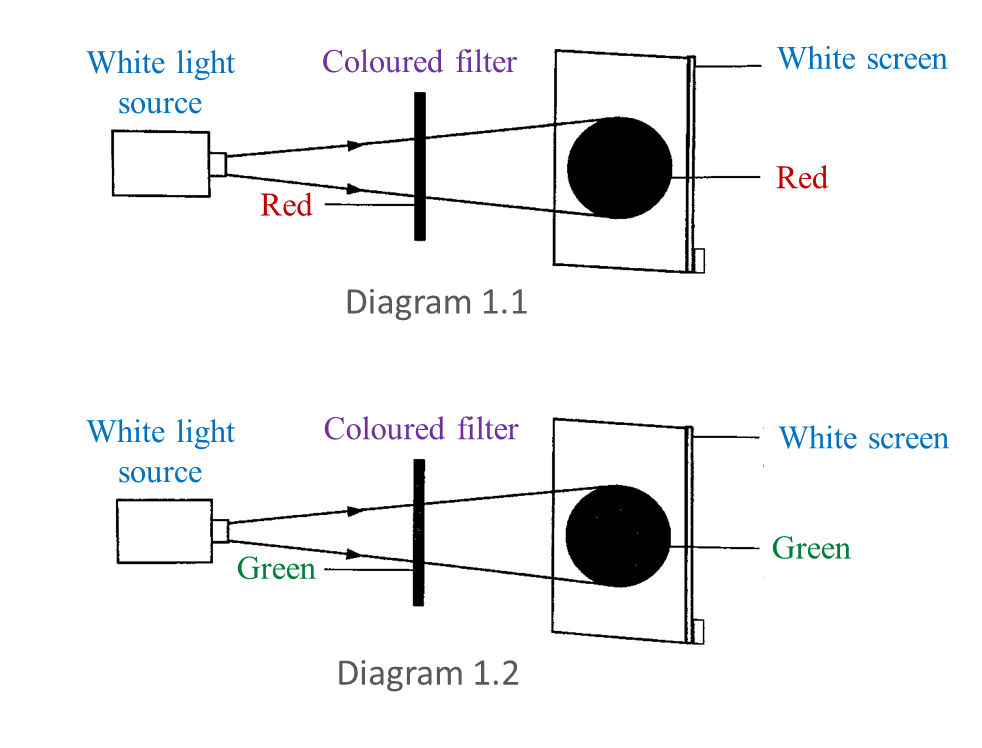(a) Based on this experiment, state the colour of light observed on the white screen. Write down your answer in Table below. [2 marks]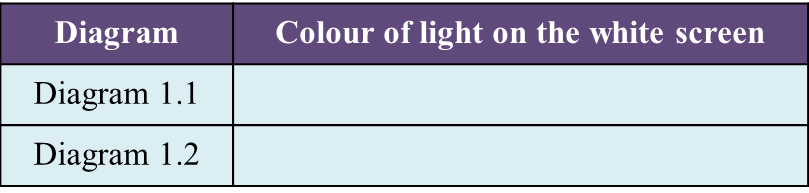(b) Write down one hypothesis for this experiment. [1 mark]

(c) State one manipulated variable in this experiment. [1 mark]

(d) Predict the colour of light observed on the white screen if blue filter is used. [1 mark]

(a)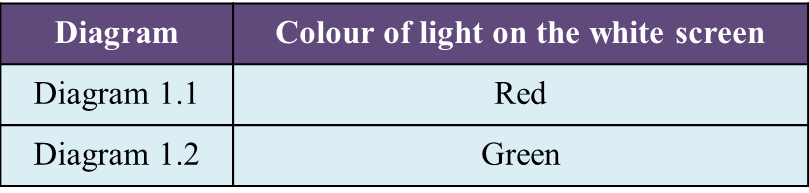(b)
The colour on the screen is the same as the colour of the filter.

(c)
The type of colour filter.

(d)
Blue

Question 2:
Diagram 2.1 shows three coloured lights projected on a white screen.(a) Based on the coloured lights labelled in Diagram 2.1, state
(i) a primary colour, [1 mark]
(ii) secondary colour. [1 mark]

(b) What is the colour represented by K? [1 mark]

(c) Diagram 2.2 shows a white light projected through a glass prism. [2 marks]
In Diagram 2.2, write down the colours of lights P and Q.(d) A green filter is placed as shown in Diagram 2.3.What colour is seen on the white screen? [1 mark]

(a)(i)
Blue/ Red/ Green

(a)(ii)
Yellow

(b)
White

(c)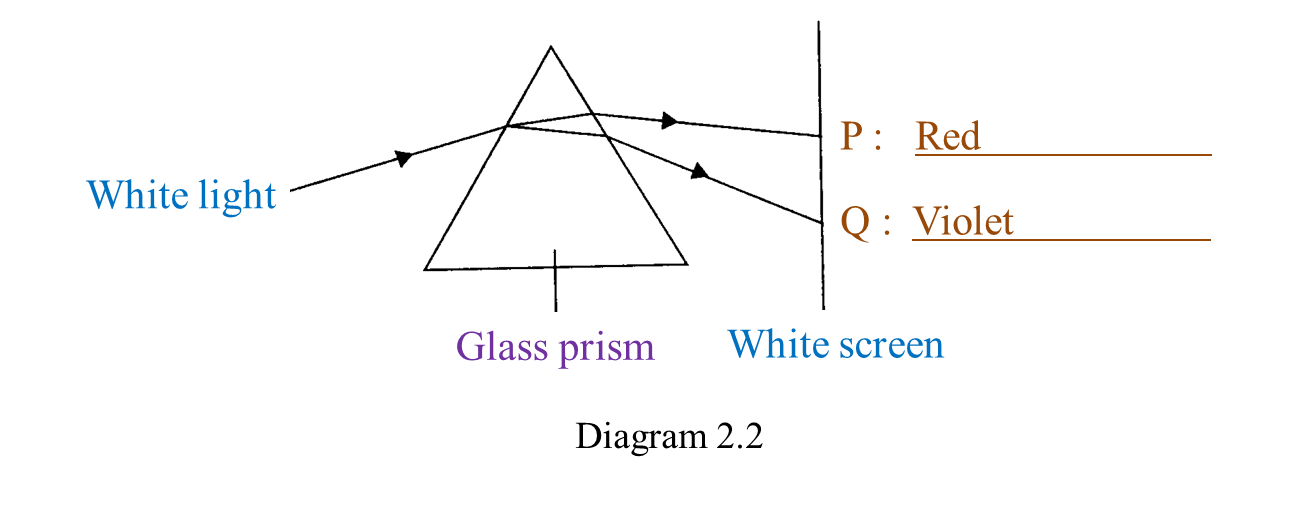(d) Green

# 7.10.1 The Formation of Images by Plane Mirrors and Lenses (Structured Questions)

Question 1:
Diagram 1.1 and Diagram 1.2 show an experiment to measure the focal length of two convex lenses.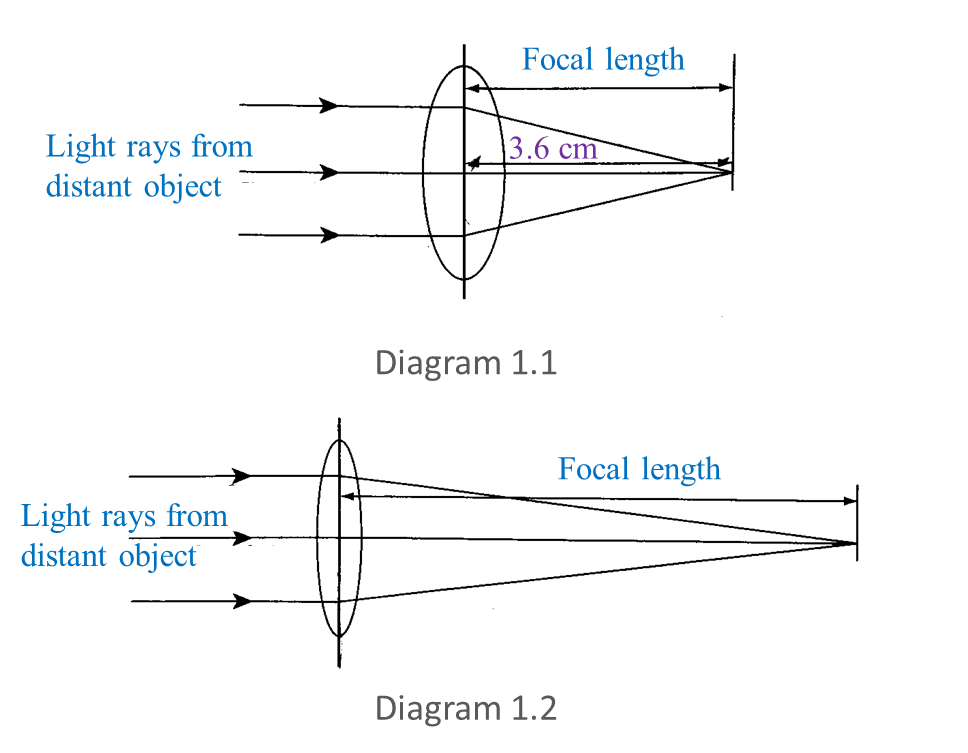(a) Measure and write down the focal length in Diagram 1.1. [1 mark]

(b) Observe Diagram 1.1 and 1.2.
What is the difference between the focal lengths of the two convex lenses? [1 mark]

(c) State one inference for this experiment. [1 mark]

(d) State the variables in this experiment.
(i) Constant variable [1 mark]
(ii) Manipulated variable [1 mark]